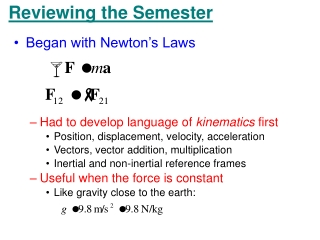Download PresentationReviewing the Semester

# Reviewing the Semester

Download Presentation## Reviewing the Semester

- - - - - - - - - - - - - - - - - - - - - - - - - - - E N D - - - - - - - - - - - - - - - - - - - - - - - - - - -
##### Presentation Transcript

1. Reviewing the Semester • Began with Newton’s Laws • Had to develop language of kinematics first • Position, displacement, velocity, acceleration • Vectors, vector addition, multiplication • Inertial and non-inertial reference frames • Useful when the force is constant • Like gravity close to the earth:

2. Reviewing the Semester • Non-constant forces • Integrating the force over space (line integral) leads to work and energy • For some forces, integral doesn’t depend on the path, just the end points; then we define the potential energy

3. Reviewing the Semester • Non-constant forces • Integrating the force over time leads to momentum • Momentum allows us to deal with systems of particles because we can add all the momenta together • Newton’s 3rd Law allows us to neglect all the internal forces and treat the system as one object

4. Reviewing the Semester • Systems of Particles • A rigid system of particles (and any other) can not only translate (momentum) but rotate • To deal with this, we developed angular kinematics and dynamics

5. Reviewing the Semester • Conservation Laws • Energy, momentum, angular momentum aren’t just useful ways to deal with Newton’s Laws • They have a deep significance because they are conserved • Conservation laws are related to symmetries in nature • Momentum Conservation is related to translational symmetries (invariance, physics doesn’t depend on our choice of origin) • Energy Conservation is related to time invariance • Angular Momentum Conservation is related to angular invariance

6. Reviewing the Semester • Applications of Newton’s Laws: Universal Gravitation • Central Force Motion

7. Reviewing the Semester • Applications of Newton’s Laws: Universal Gravitation • Kepler’s Laws • Planets orbit in ellipses with sun at one focus • Planets sweep out equal areas in equal times: angular momentum conserved • Period-Orbit Size relationship

8. Reviewing the Semester • Applications of Newton’s Laws: Simple Harmonic Motion • For systems where Hooke’s Law applies • Pendulums

9. Reviewing the Semester • Applications of Newton’s Laws: Simple Harmonic Motion • When damping and/or forcing are present

10. Reviewing the Semester • Applications of Newton’s Laws: Waves • Waves obey the wave equation • Transverse waves on a string • Harmonic solutions Don’t forget energy and power transmission!

11. Reviewing the Semester • Applications of Newton’s Laws: Waves • Sound waves • Pressure vs displacement • Decibels • Doppler Shift

12. Reviewing the Semester • Applications of Newton’s Laws: Waves • Standing waves • On a string • In an air column (closed at one end) • Interference • Waves of the same frequency but different phases • Constructive or destructive • Beats • Interference in time • Beat frequency is difference between two wave freq.

13. Reviewing the Semester • Statistical Mechanics and Thermodynamics • Can’t follow each molecule explicitly • Average energy, temperature • State Equation for Ideal Gas

14. Reviewing the Semester • Statistical Mechanics and Thermodynamics • Boltzmann Distribution • Probability for a given velocity (a vector!) goes as its energy • Maxwell-Boltzmann Velocity distribution • Probability to have a given magnitude for the velocity • More ways to make a big velocity than small

15. Reviewing the Semester • Statistical Mechanics and Thermodynamics • 1st Law of Thermodynamics • Processes • Isochoric • Isobaric • Isothermal • Adiabatic

16. Reviewing the Semester • Statistical Mechanics and Thermodynamics • 2nd Law of Thermodynamics • Can’t have heat flowing cold-to-hot without work • Can’t have heat changing completely to work without some being lost • Heat Engines • Work is area enclosed in cycle (on PV diagram) • Carnot cycle (isothermal & adiabatic) most efficient • Sterling cycle (isothermal & ischoric) • Equipartition Theorem